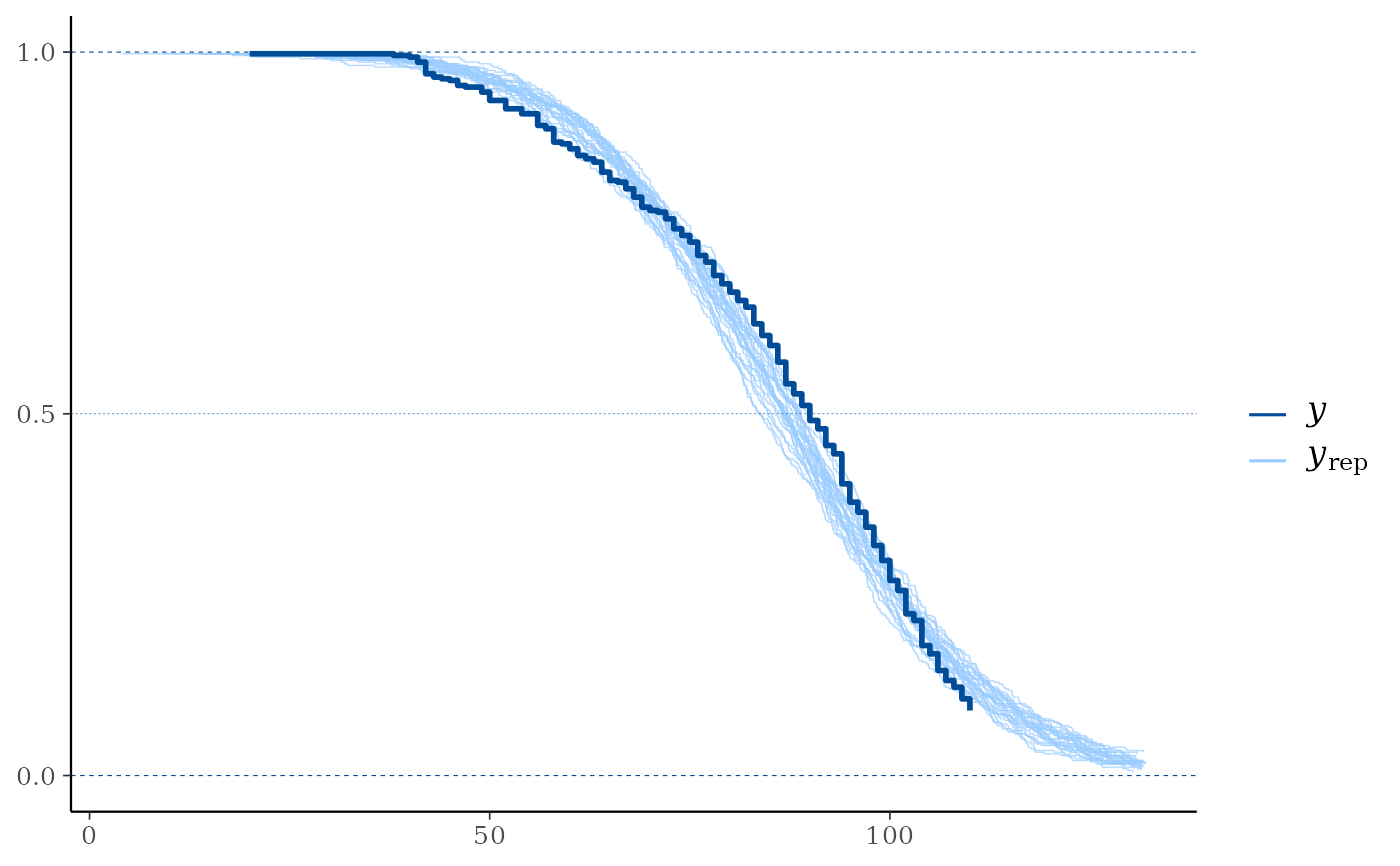Compare the empirical distribution of censored data y to the distributions of simulated/replicated data yrep from the posterior predictive distribution. See the Plot Descriptions section, below, for details.

Although some of the other bayesplot plots can be used with censored data, ppc_km_overlay() is currently the only plotting function designed specifically for censored data. We encourage you to suggest or contribute additional plots at github.com/stan-dev/bayesplot.

ppc_km_overlay(y, yrep, ..., status_y, size = 0.25, alpha = 0.7)

## Arguments

y A vector of observations. See Details. An $$S$$ by $$N$$ matrix of draws from the posterior predictive distribution, where $$S$$ is the size of the posterior sample (or subset of the posterior sample used to generate yrep) and $$N$$ is the number of observations (the length of y). The columns of yrep should be in the same order as the data points in y for the plots to make sense. See Details for additional instructions. Currently unused. The status indicator for the observations from y. This must be a numeric vector of the same length as y with values in {0, 1} (0 = right censored, 1 = event). Passed to the appropriate geom to control the appearance of the yrep distributions.

## Value

A ggplot object that can be further customized using the ggplot2 package.

## Plot Descriptions

ppc_km_overlay()

Empirical CCDF estimates of each dataset (row) in yrep are overlaid, with the Kaplan-Meier estimate (Kaplan and Meier, 1958) for y itself on top (and in a darker shade). This is a PPC suitable for right-censored y. Note that the replicated data from yrep is assumed to be uncensored.

Gelman, A., Carlin, J. B., Stern, H. S., Dunson, D. B., Vehtari, A., and Rubin, D. B. (2013). Bayesian Data Analysis. Chapman & Hall/CRC Press, London, third edition. (Ch. 6)

Kaplan, E. L. and Meier, P. (1958). Nonparametric estimation from incomplete observations. Journal of the American Statistical Association. 53(282), 457--481. doi:10.1080/01621459.1958.10501452.

Other PPCs: PPC-discrete, PPC-distributions, PPC-errors, PPC-intervals, PPC-loo, PPC-overview, PPC-scatterplots, PPC-test-statistics

## Examples

color_scheme_set("brightblue")
y <- example_y_data()
# For illustrative purposes, (right-)censor values y > 110:
status_y <- as.numeric(y <= 110)
y <- pmin(y, 110)
# In reality, the replicated data (yrep) would be obtained from a
# model which takes the censoring of y properly into account. Here,
# for illustrative purposes, we simply use example_yrep_draws():
yrep <- example_yrep_draws()
dim(yrep)#>  500 434# \donttest{
ppc_km_overlay(y, yrep[1:25, ], status_y = status_y)# }